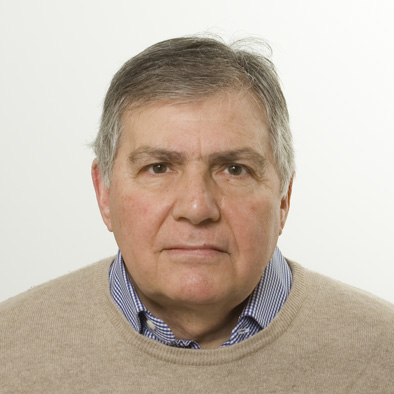# Computational cardiac electrophysiology: motivations, mathematical models and scalable solvers#### Keywords

Computational Medicine for the Cardiocirculatory System
Speaker:
Piero Colli Franzone
Affiliation:
Università di Pavia, Dipartimento di Matematica
When:
Thursday 20th April 2017
Time:
14:00:00
Where:
Aula Seminari 'Saleri' VI Piano MOX-Dipartimento di Matematica, Politecnico di Milano - Edificio 14
Abstract:
Life sciences could benefit immensely from the massive growth of HPC processing power occurred in the last ten years. Indeed, complex biological systems are described by sophisticated mathematical models, whose solution requires highly scalable solvers. In particular, for what concerns cardiac electrophysiology, the simulation of the electrical excitation of the heart muscle, and the subsequent contraction-relaxation process, represents a challenging computational task. In the present talk, we will describe the main mathematical model of the cardiac electrical and mechanical interactions, the so-called cardiac electro-mechanical coupling model. This model consists of a system of non-linear partial differential equations (PDEs), constituted by four sub-models: the anisotropic Bidomain model, i.e. a system of degenerate parabolic reaction-diffusion PDEs describing the electrical current flow through the tissue; the membrane model of the cardiac cell myocyte describing the ionic fluxes across the membrane, including stretch-activated channels; the active tension model describing the intracellular calcium dynamics and the consequent generation of the cellular force; the quasi-static anisotropic finite elasticity equations describing the deformation of the ventricular walls. The complex interaction between the cardiac bioelectrical and mechanical phenomena is modeled by a system of non-linear partial differential equations (PDEs), known as cardiac electromechanical coupling (EMC) model. Due to the extremely different spatial and temporal scales of the physical phenomena occurring during a single heartbeat, the discretization of the EMC model with finite elements in space and finite differences in time yields the solution of thousands of large scale linear systems, with O(106 - 108) degrees of freedom each. The effective solution of such linear systems requires the use of hundreds/thousands processors and, consequently, of highly scalable preconditioners. The numerical simulations are based on our finite element parallel solver, which employs Multilevel Additive Schwarz preconditioners for the solution of linear systems arising from the discretization of the Bidomain equations and Newton-Krylov-Algebraic Multigrid methods for the solution of the non-linear systems arising from the discretization of the finite elasticity equations. Finally, the results of three-dimensional parallel simulations will demonstrate the effectiveness of the resulting algorithms. contact: christian.vergara@polimi.it
Note:
The topic of his research are control problems, boundary integral methods, reaction-diffusion systems, Eikonal equations, homogenization. In the field of Mathematical Modeling and Scientific Computation applied to Electrocardiology is a renowned expert for his contributions in the Electrocardiographic inverse problem, in the propagation phenomena in excitable media and in large scale simulations of the myocardial activation